# Search by Topic

Filter by: Content type:
Age range:
Challenge level:

There are 33 NRICH Mathematical resources connected to Algorithms, you may find related items under Decision Mathematics and Combinatorics.

Broad Topics > Decision Mathematics and Combinatorics > Algorithms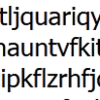### Probably a Code?

##### Age 16 to 18 Challenge Level:

Is the regularity shown in this encoded message noise or structure?##### Age 7 to 14 Challenge Level:

Watch our videos of multiplication methods that you may not have met before. Can you make sense of them?### Funny Factorisation

##### Age 11 to 14 Challenge Level:

Using the digits 1 to 9, the number 4396 can be written as the product of two numbers. Can you find the factors?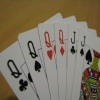### Sorted

##### Age 14 to 18 Challenge Level:

How can you quickly sort a suit of cards in order from Ace to King?### Ip?

##### Age 16 to 18 Challenge Level:

Seventh challenge cipher### Jluuis or Even Asutguus?

##### Age 16 to 18 Challenge Level:

Sixth challenge cipher### Up a Semitone?

##### Age 16 to 18 Challenge Level:

Fifth challenge cipher### Semicircle

##### Age 16 to 18 Challenge Level:

Fourth challenge cipher### Vital?

##### Age 16 to 18 Challenge Level:

Third challenge cipher### A Fine Thing?

##### Age 16 to 18 Challenge Level:

Second challenge cipher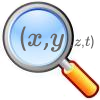### Stage 5 Cipher Challenge

##### Age 16 to 18 Challenge Level:

Can you crack these very difficult challenge ciphers? How might you systematise the cracking of unknown ciphers?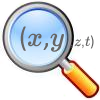### The Best Square

##### Age 14 to 18 Challenge Level:

How would you judge a competition to draw a freehand square?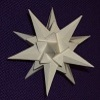### Happy Birthday

##### Age 16 to 18 Challenge Level:

Can you interpret this algorithm to determine the day on which you were born?### An Introduction to Computer Programming and Mathematics

##### Age 16 to 18

This article explains the concepts involved in scientific mathematical computing. It will be very useful and interesting to anyone interested in computer programming or mathematics.### Peaches in General

##### Age 14 to 16 Challenge Level:

It's like 'Peaches Today, Peaches Tomorrow' but interestingly generalized.### Zeller's Birthday

##### Age 14 to 16 Challenge Level:

What day of the week were you born on? Do you know? Here's a way to find out.### Unusual Long Division - Square Roots Before Calculators

##### Age 14 to 16 Challenge Level:

However did we manage before calculators? Is there an efficient way to do a square root if you have to do the work yourself?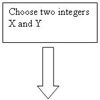### Procedure Solver

##### Age 16 to 18 Challenge Level:

Can you think like a computer and work out what this flow diagram does?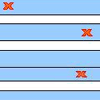### Stretching Fractions

##### Age 14 to 16 Challenge Level:

Imagine a strip with a mark somewhere along it. Fold it in the middle so that the bottom reaches back to the top. Stetch it out to match the original length. Now where's the mark?### On What Day Did it Happen?

##### Age 5 to 14

Read this article to find out the mathematical method for working out what day of the week each particular date fell on back as far as 1700.### Tournament Scheduling

##### Age 11 to 16

Scheduling games is a little more challenging than one might desire. Here are some tournament formats that sport schedulers use.### Divided Differences

##### Age 16 to 18

When in 1821 Charles Babbage invented the Difference Engine' it was intended to take over the work of making mathematical tables by the techniques described in this article.### X Marks the Spot

##### Age 11 to 14 Challenge Level:

When the number x 1 x x x is multiplied by 417 this gives the answer 9 x x x 0 5 7. Find the missing digits, each of which is represented by an "x" .### Tis Unique

##### Age 11 to 14 Challenge Level:

This addition sum uses all ten digits 0, 1, 2...9 exactly once. Find the sum and show that the one you give is the only possibility.### Kids

##### Age 11 to 14 Challenge Level:

Find the numbers in this sum### Slippy Numbers

##### Age 11 to 14 Challenge Level:

The number 10112359550561797752808988764044943820224719 is called a 'slippy number' because, when the last digit 9 is moved to the front, the new number produced is the slippy number multiplied by 9.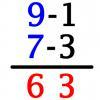### Vedic Sutra - All from 9 and Last from 10

##### Age 14 to 16 Challenge Level:

Vedic Sutra is one of many ancient Indian sutras which involves a cross subtraction method. Can you give a good explanation of WHY it works?### Medal Muddle

##### Age 11 to 14 Challenge Level:

Countries from across the world competed in a sports tournament. Can you devise an efficient strategy to work out the order in which they finished?### Alphabet Soup

##### Age 11 to 14 Challenge Level:

This challenge is to make up YOUR OWN alphanumeric. Each letter represents a digit and where the same letter appears more than once it must represent the same digit each time.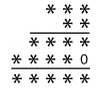### Long Multiplication

##### Age 11 to 14 Challenge Level:

A 3 digit number is multiplied by a 2 digit number and the calculation is written out as shown with a digit in place of each of the *'s. Complete the whole multiplication sum.### Skeleton

##### Age 11 to 14 Challenge Level:

Amazing as it may seem the three fives remaining in the following skeleton' are sufficient to reconstruct the entire long division sum.### Triangle Incircle Iteration

##### Age 14 to 16 Challenge Level:

Keep constructing triangles in the incircle of the previous triangle. What happens?### Route to Root

##### Age 16 to 18 Challenge Level:

A sequence of numbers x1, x2, x3, ... starts with x1 = 2, and, if you know any term xn, you can find the next term xn+1 using the formula: xn+1 = (xn + 3/xn)/2 . Calculate the first six terms of this. . . .